Problem Statement:

You are an engineer trying to decide between propeller propulsion and turbojet propulsion to power an airplane. Your analysis to this point has determined the following parameters for each engine design at the desired operating conditions:

 Propeller Turbojet Ae (m2) 1.16 0.059 ρe (kg/m3) 1.15 0.52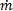(kg/s) 80.0 10.8

Wind tunnel analysis of the airframe design indicates that the drag coefficient times the cross-sectional area of the airplane is CDA = 1.67 m2. For simplicity, we will assume CDA is the same for both engine types. Find the cruise speed and propulsive efficiency of your aircraft for each engine type. Which engine is best?

Note: When computing drag you may assume the air density is ρ = 1.15 kg/m3.

Solution:

Using the equation for, we can find the jet velocity corresponding to each design: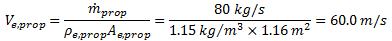Using the same approach, we find Ve,jet = 352 m/s. Then, using the fact that drag and thrust balance we can equate the expressions used to compute thrust and drag to obtain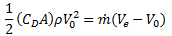Substituting the known values of CDA, ρ,, and Ve for configuration and rearranging we have

Propeller: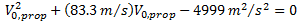Jet: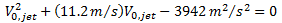We can solve for V0 using the quadratic formula. For the propeller case this gives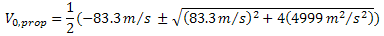Since V0 must be positive, we take the "+" solution, giving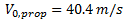Similarly, we have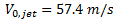for the jet case.

Now that we know Ve and V0 we can compute propulsive efficiency from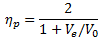Using the above results we get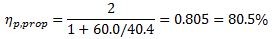And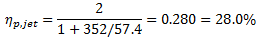Summary:

V0 (m/s) ηp (%) 40.4 80.5 57.4 28.0

The turbojet gives a much higher airplane speed (V0), but its propulsive efficiency is much smaller. The turbojet engine produces a small diameter, high velocity jet, but it also waists a lot of the kinetic energy given to the jet, resulting in a lower propulsive efficiency. The turbojet used in this example is a bit small and larger engines can perform better, but generally propellers tend to have higher ηp and lower V0 than turbojets.

So which is better, the propeller or the turbojet? That depends on what you want. Propellers tend to be more efficient while turbojets will get you there faster. Here we only computed propulsive efficiency, but the overall efficiencies of propellers tend to be higher as well since the two often have comparable thermal efficiencies. Turboprops and turbofans provide a range of compromising options on the speed and efficiency spectra where generally efficiency is traded for speed.

Other factors to consider when choosing an engine are cost, maintenance, and matching the engine with the aircraft design. A propeller engine driven by an internal combustion engine can have lower cost and maintenance than a turbojet (or turboprop for that matter), but it may not be powerful enough to propel the aircraft at the desired speed. Proper selection of an engine requires an in-depth knowledge of propulsion systems and airframes!